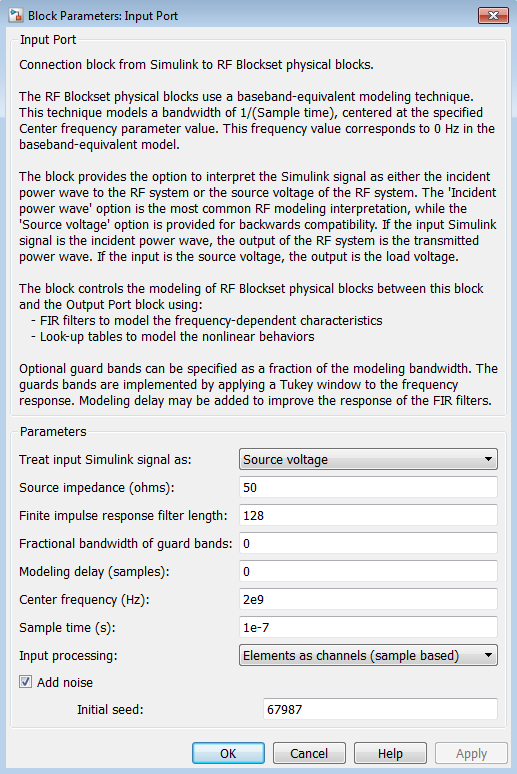## Determine Modeling Frequencies

When you simulate an RF model, the Output Port block uses Input Port block parameters to determine the modeling frequencies f for the physical system that is bracketed between the Input Port block and the Output Port block. f is an N-element vector, where N is the finite impulse response filter length. The modeling frequencies are a function of the center frequency fc and the sample time ts. The following figure shows the Input Port block parameters that determine the modeling frequencies.fn is the nth element of the vector of modeling frequencies, f, and is given by

`$\begin{array}{cc}{f}_{n}={f}_{\mathrm{min}}+\frac{n-1}{{t}_{s}N}& n=1,...,N\end{array}$`

where

`${f}_{\mathrm{min}}={f}_{c}-\frac{1}{2{t}_{s}}$`